##### Microeconomics For Dummies - UK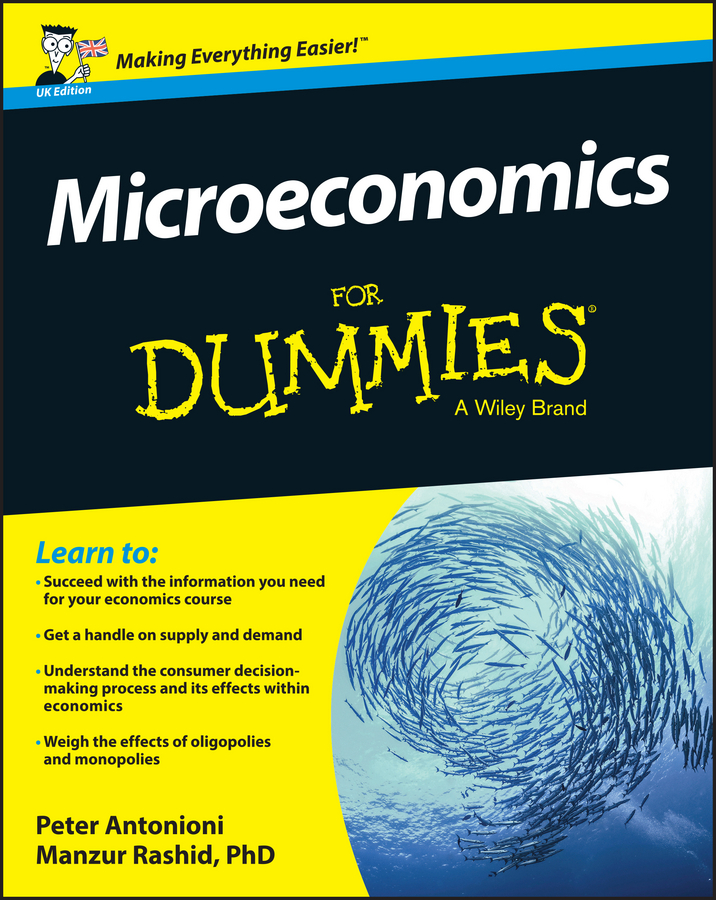You can use calculus to maximize the total profit equation. Because total revenue and total cost are both expressed as a function of quantity, you determine the profit-maximizing quantity of output by taking the derivative of the total profit equation with respect to quantity, setting the derivative equal to zero, and solving for the quantity.

The market demand curve for the good your monopoly produces is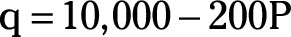where q is the market and firm’s quantity demanded, and P is the market price in dollars.

Using the demand equation to derive total revenue as a function of q requires the following steps:

1. Add 200P to both sides of the demand equation.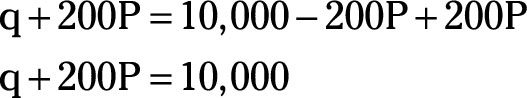2. Subtract q from both sides of the equation.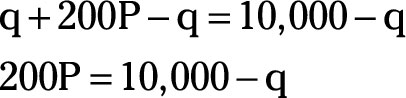3. Divide both sides of the equation by 200.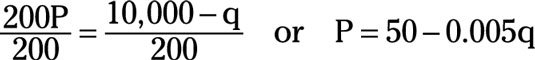4. To determine total revenue, multiply both sides of the demand equation by q.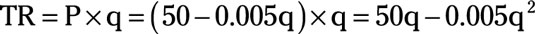This equation tells you how much total revenue equals given any value for quantity, q. Thus, total revenue is a function of q.

If your total cost equation is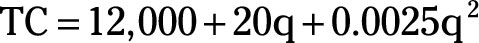total profit, ð, is determined by subtracting total cost from total revenue, or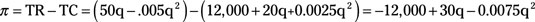After you have the total profit equation, the following steps enable you to determine the profit-maximizing quantity and price:

1. Take the derivative of the total profit equation with respect to quantity.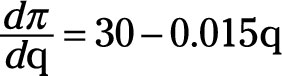2. Set the derivative equal to zero and solve for q.

This is your profit-maximizing quantity of output.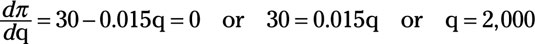3. Substitute the profit-maximizing quantity of 2,000 into the demand equation and solve for P.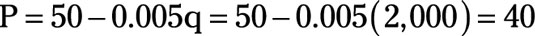Or you should set a price of \$40 for the good.

4. Finally, total profit is determined by substituting 2,000 for q in the total-profit equation.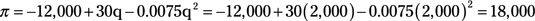Your total profit equals \$18,000.